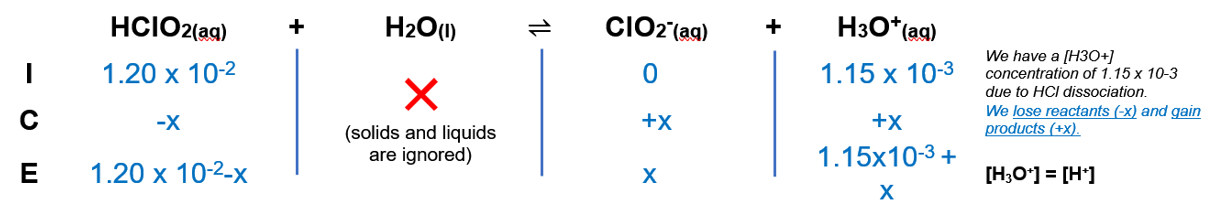# Problem: Calculate the pH of a solution that is 1.15×10−3 M in HCl and 1.20×10−2 M in HClO2.

###### FREE Expert Solution

We’re being asked to calculate the pH of a solution that is 1.15×10−3 M in HCl and 1.20×10−2M in HClO2.

HClO2 is an oxyacid, and in order to be considered strong, there must be 2 or more oxygens than Hydrogens.

HClO2 having only one more oxygen than hydrogen is not enough to make it a strong acid, therefore it a weak.

Remember that weak acids partially dissociate in water and that acids donate H+ to the base (water in this case):

The dissociation of HClO2 is as follows.

HClO2(aq) + H2O(l)  H3O+(aq) + ClO2(aq) Ka = 1.1 × 10–2

HCl is a strong acid. It dissociates completely in aqueous solution with HClO2

HCl(aq)    →         H(aq)       +      Cl – (aq)

After dissociation    (1.15 x 10-3)    (1.15 x 10-3)

That is why initially, we already have a [H3O+] concentration of 1.15 x 10-3 M.

From this, we can construct an ICE table. Remember that liquids are ignored in the ICE table.The Ka expression for HClO2 is:

Liquids are ignored in the Kexpression.

Note that each concentration is raised by the stoichiometric coefficient: [HClO2], [H3O+] and [ClO2] are raised to 1. We also have a common ion from the 1.15 x 10-3 M HCl which is [H3O+].

81% (96 ratings)###### Problem Details

Calculate the pH of a solution that is 1.15×10−3 M in HCl and 1.20×10−2 M in HClO2.